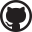# Poleiro

## Where the Coq lives

| Comments# Summing combinatorial games

In the previous post, we've introduced the concept of combinatorial game and saw how we can employ a simple formalism to model all such games uniformly, the game type. To see what this point of view can bring us, we'll discuss the idea of summing combinatorial games, which can be used to analyze games by decomposing them into smaller, simpler ones. As we'll see, this notion has a straightforward computational interpretation for the game type, making it convenient to manipulate and reason about.
The intuition behind summing games is that they often evolve to a point where they can be seen as several smaller ones being played "simultaneously". In go, for instance, the board is progressively partioned into different regions, and each player must choose in which of those to play during their turn. This suggests a representation where the state of a game is given by the product of the states of the subgames. To make a move, a player modifies the state of one of the subgames according to its rules, leaving the rest of the state untouched.
To define the sum of two combinatorial games (as given by the combinatorial_game type), we can simply combine the lists of moves available for each game component. We must still show that this results in a terminating game, but it suffices to observe that the valid_move relation in this case is equivalent to the product of two well-founded relations, and the Coq standard library provides lemmas for dealing with this case.

Definition cg_pair_order {cg1 cg2} :=
symprod _ _ (valid_move cg1) (valid_move cg2).
Definition cg_pair_order_wf {cg1 cg2} : well_founded cg_pair_order :=
wf_symprod _ _ _ _ (finite_game cg1) (finite_game cg2).

Program Definition sum_cg (cg1 cg2 : combinatorial_game)
: combinatorial_game :=
{| position := position cg1 * position cg2;
moves p pos := map (fun pos1' => (pos1', snd pos))
(moves cg1 p (fst pos)) ++
map (fun pos2' => (fst pos, pos2'))
(moves cg2 p (snd pos)) |}.
Next Obligation.
match goal with
| |- well_founded ?R =>
assert (EQ : RelationClasses.relation_equivalence R cg_pair_order)
end.
(* ... *)
rewrite EQ.
apply cg_pair_order_wf.
Qed.

A problem with this definition is that it is not directly amenable to computation. We can overcome this problem by defining game sums directly over the game type. Since game is universal, we can hope this should be enough to define what a sum of games is generically. A naive adaptation doesn't work, as recursive calls to sum don't have a single decreasing argument, e.g.

Fail Fixpoint sum (g1 g2 : game) : game :=
Game (map_game game_as_cg g1 Left (fun g1' P => sum g1' g2) ++
map_game game_as_cg g2 Left (fun g2' P => sum g1 g2'))
(map_game game_as_cg g1 Right (fun g1' P => sum g1' g2) ++
map_game game_as_cg g2 Right (fun g2' P => sum g1 g2')).
(* Error: Cannot guess decreasing argument of fix. *)

One solution is again to pair both arguments and use the Fix combinator with cg_pair_order_wf. Manipulating proof terms in the recursive calls can be made less awkward by using the simple refine tactic:

Definition sum (g1 g2 : game) : game.
simple refine (
Fix cg_pair_order_wf (fun _ => game)
(fun gs =>
match gs with
| (g1, g2) => fun sum =>
let sum_fst g1' P := sum (g1', g2) _ in
let sum_snd g2' P := sum (g1, g2') _ in
Game (map_game game_as_cg g1 Left sum_fst ++
map_game game_as_cg g2 Left sum_snd)
(map_game game_as_cg g1 Right sum_fst ++
map_game game_as_cg g2 Right sum_snd)
end) (g1, g2));
clear - P; constructor; trivial.
Defined.

As with all definitions involving Fix, we must now prove a lemma that shows how sum unfolds. The proof is very similar to the one of embed_in_game_eq, and is thus omitted.

Lemma sum_eq (g1 g2 : game) :
sum g1 g2 =
Game (map (fun g1' => sum g1' g2) (left_moves g1) ++
map (fun g2' => sum g1 g2') (left_moves g2))
(map (fun g1' => sum g1' g2) (right_moves g1) ++
map (fun g2' => sum g1 g2') (right_moves g2)).

The name sum suggests that combinatorial games could behave like numbers. We won't discuss this correspondence in much detail for now, but some interesting identities do show up already:

Lemma zero_plus_zero : sum zero zero = zero.

Lemma one_plus_zero : sum one zero = one.

Showing that sum correctly computes what it should is not difficult: we proceed by well-founded induction combining simple lemmas about the behavior of sum, embed_in_game, and map. We need an auxiliary result that allows us to apply our inductive hypothesis:

Lemma map_ext_strong :
forall {A B}
(l : list A)
(f g : A -> B)
(EXT : forall x, In x l -> f x = g x),
map f l = map g l.

The statement of lemma just says that embed_in_game exchanges sum and sum_cg.

Lemma sum_is_sum (cg1 cg2 : combinatorial_game)
(pos1 : position cg1) (pos2 : position cg2) :
embed_in_game (sum_cg cg1 cg2) (pos1, pos2) =
sum (embed_in_game cg1 pos1) (embed_in_game cg2 pos2).
Proof.
remember (pos1, pos2) as pos.
replace pos1 with (fst pos) by (destruct pos; simpl; congruence).
replace pos2 with (snd pos) by (destruct pos; simpl; congruence).
clear.
induction pos as [[pos1 pos2] IH]
using (well_founded_ind cg_pair_order_wf).
rewrite embed_in_game_eq, sum_eq. simpl.
repeat rewrite (embed_in_game_moves _ _ Left).
repeat rewrite (embed_in_game_moves _ _ Right).
repeat rewrite map_app. repeat rewrite map_map.
do 2 f_equal;
apply map_ext_strong; intros pos IN; apply IH; constructor; eexists; eauto.
Qed.

In the next posts, we will see how to use this machinery when decomposing games as sums and comparing subgames.